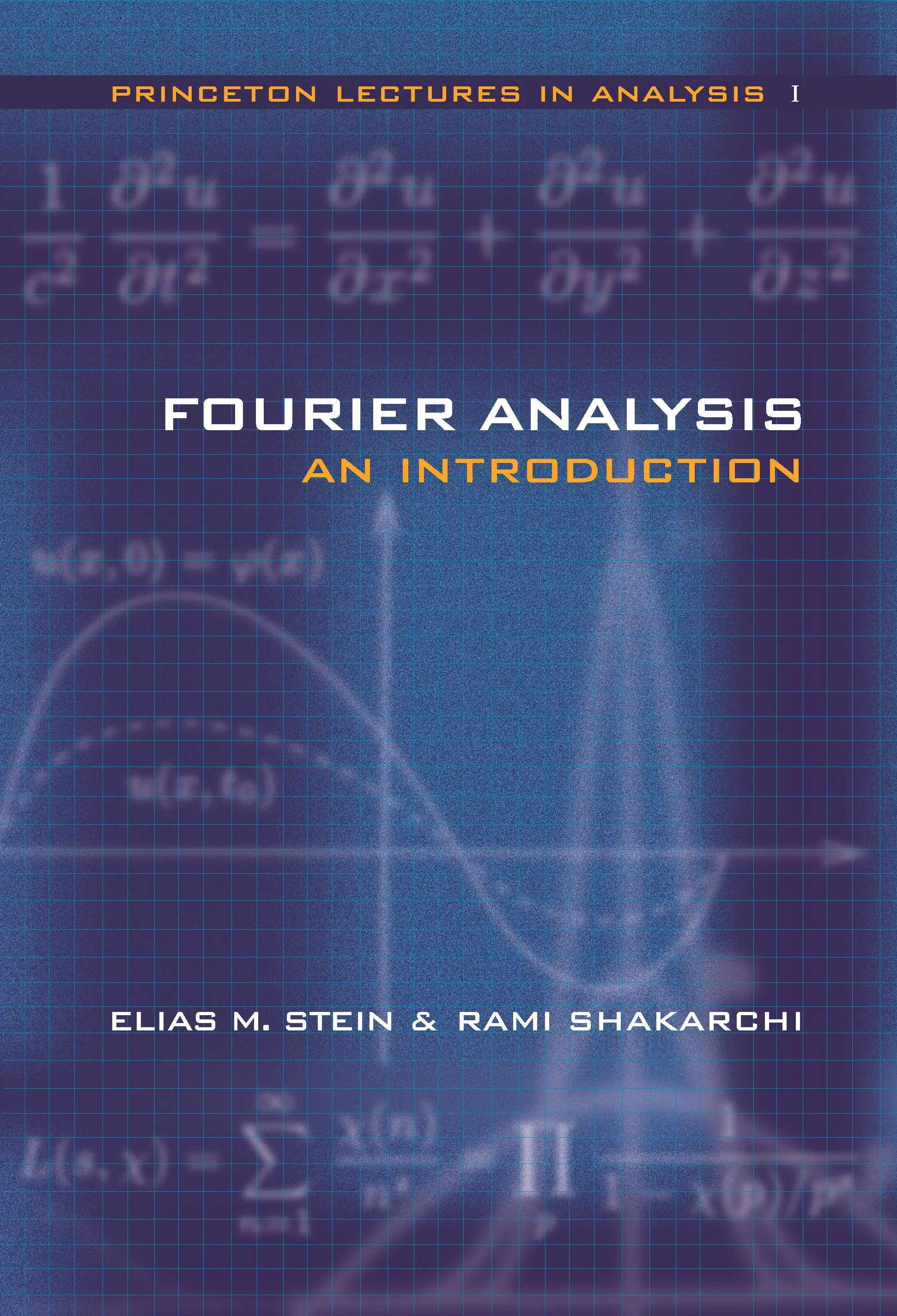#### Fourier Analysis

Elias M. Stein

##### ebooks

Many of our ebooks are available for purchase from these online vendors:

Many of our ebooks are available through library electronic resources including these platforms:

# Fourier Analysis: An Introduction## ebook

ISBN:
Published:
Feb 11, 2011
2003
Illus:
40 line illus.

This first volume, a three-part introduction to the subject, is intended for students with a beginning knowledge of mathematical analysis who are motivated to discover the ideas that shape Fourier analysis. It begins with the simple conviction that Fourier arrived at in the early nineteenth century when studying problems in the physical sciences — that an arbitrary function can be written as an infinite sum of the most basic trigonometric functions.

The first part implements this idea in terms of notions of convergence and summability of Fourier series, while highlighting applications such as the isoperimetric inequality and equidistribution. The second part deals with the Fourier transform and its applications to classical partial differential equations and the Radon transform; a clear introduction to the subject serves to avoid technical difficulties. The book closes with Fourier theory for finite abelian groups, which is applied to prime numbers in arithmetic progression.

In organizing their exposition, the authors have carefully balanced an emphasis on key conceptual insights against the need to provide the technical underpinnings of rigorous analysis. Students of mathematics, physics, engineering and other sciences will find the theory and applications covered in this volume to be of real interest.

The Princeton Lectures in Analysis represents a sustained effort to introduce the core areas of mathematical analysis while also illustrating the organic unity between them. Numerous examples and applications throughout its four planned volumes, of which Fourier Analysis is the first, highlight the far-reaching consequences of certain ideas in analysis to other fields of mathematics and a variety of sciences. Stein and Shakarchi move from an introduction addressing Fourier series and integrals to in-depth considerations of complex analysis; measure and integration theory, and Hilbert spaces; and, finally, further topics such as functional analysis, distributions and elements of probability theory.